# 5 Cool Math Tricks for the Classroom That Make Numbers ClickLet’s face it: Our students love math tricks, often more than we do as teachers.

These tricks make them feel like they’re taking a shortcut and pulling a rabbit out of a hat. Still, kids need to learn how to problem-solve, and tricks or shortcuts don’t always serve them when it comes time to work their way through a math problem.

So are there any math tricks for kids that are worth sharing? Is there any way to do so without undermining math fluency? Well, sure! There’s research out there that suggests it’s OK for kids to pick up some math tricks — and different math strategies — once they’re adept at a particular concept.

We’ve pulled together a few of our favorite math tricks for kids that are worth sharing. From tricks to make division a snap to new ways to look at subtraction, there’s a lot of packed in here. Before you teach them, might we suggest discussing when and where “tricks” are appropriate?

## Cool Math Tricks for Kids

### Divisibility Rules

OK, so these are “rules” more so than math tricks, but learning them certainly helps students make quick work of division problems, so we’ve got to get started right here!

• A number is divisible by 2 if the last digit of the one’s place is divisible by 2.
• A number is divisible by 3 if the sum of the digits is divisible by 3.
• A number is divisible by 4 if the last two digits in the number are divisible by 4.
• A number is divisible by 5 if the last digit is a 0 or 5.
• A number is divisible by 6 if the number is divisible by both 2 and 3.
• A number is divisible by 8 if the sum of the digits is divisible by 9.

### Easy Subtraction with No Grouping

Once your students have an understanding of how to do subtraction with regrouping (borrowing), this little shortcut is a great math trick to teach your students!

Introduce the concept of looking at subtraction as the distance between two numbers.

So, if you look at the numbers 4,000 and 1,394, the distance between those two numbers is the same as the distance between 3,999 and 1,393. Therefore, if you are provided with the problem 4,000-1,394, you can subtract 1 from each of those numbers and subtract 1,393 from 3,999 to get the same answer.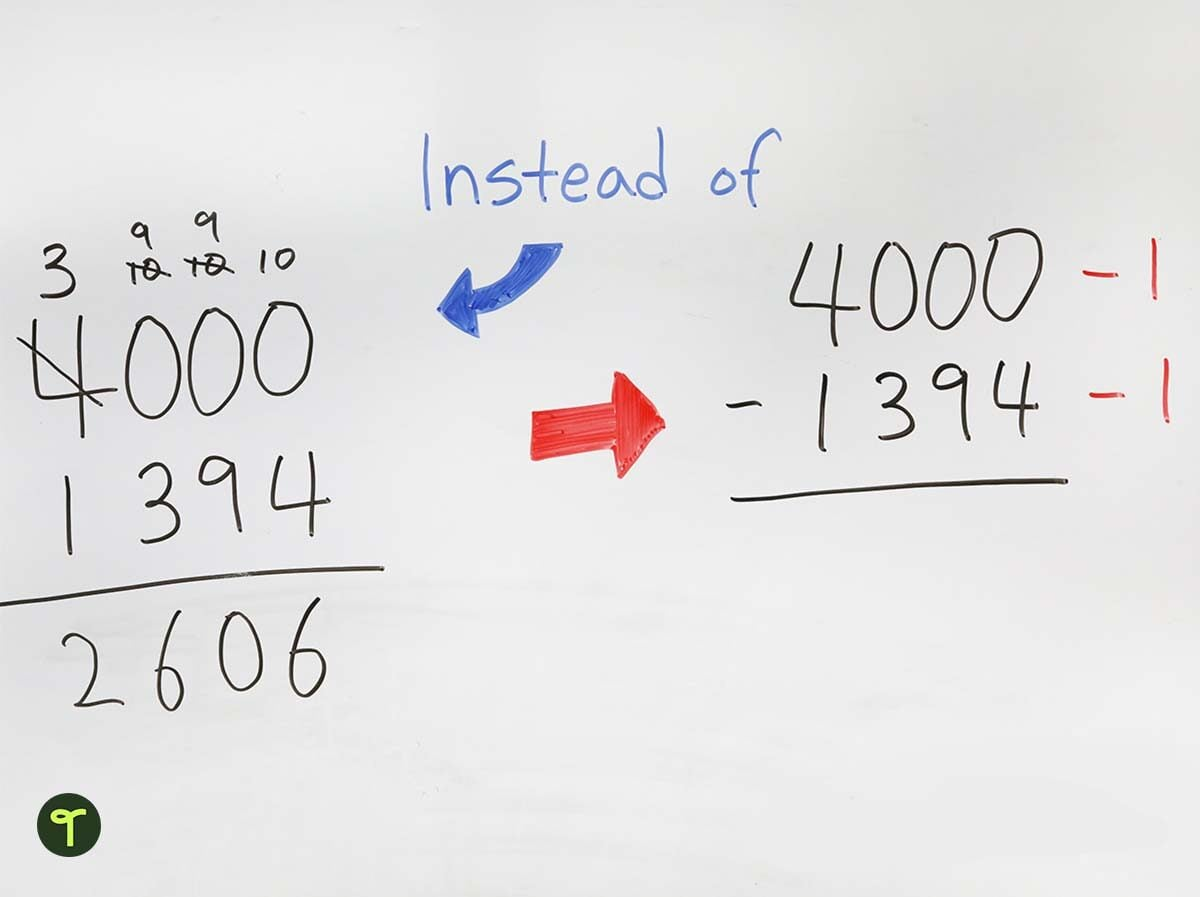Obviously, you are not going to always subtract 1 — that’s only if you need to make the top number (or most of the digits in that number) smaller to decrease the amount of ‘borrowing’ and ‘grouping’ you have to do.

A great conversation to have with your students: Discuss why or why not it is worth subtracting the 1 first. This is part of helping students make sense of what is happening, not simply memorizing a cool math trick.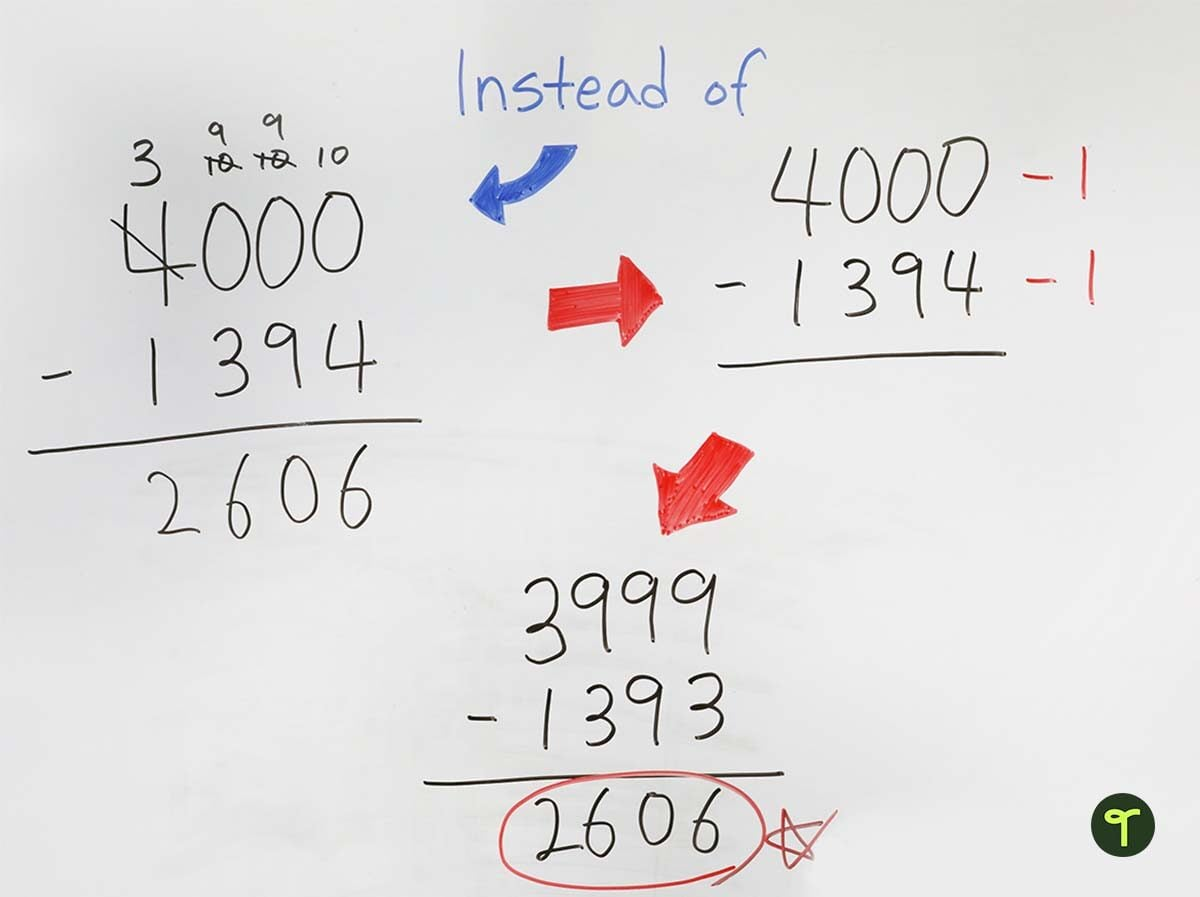Note: Some subtraction problems may not be an easy subtract 1 scenario. For example 652 – 198. Taking 1 off each of these numbers doesn’t result in an easier subtraction sum. Instead, you could simply subtract 3 to make it easier. There is still some regrouping needed however, it’s not as difficult.

Print a subtraction strategies activity that makes use of addition to help students find the answer.

### Comparing Fractions Math Trick

Fractions can be a challenge for young mathematicians, especially when students need to compare two fractions that don’t have the same denominator.

There is a really cool math trick that can help kids figure out which fraction is larger. By multiplying the top number of each fraction by the bottom number of the other fraction you can easily get the answer.

Teach Starter Teacher Tip: This is also called ‘cross multiplying’ because you create a cross in the middle.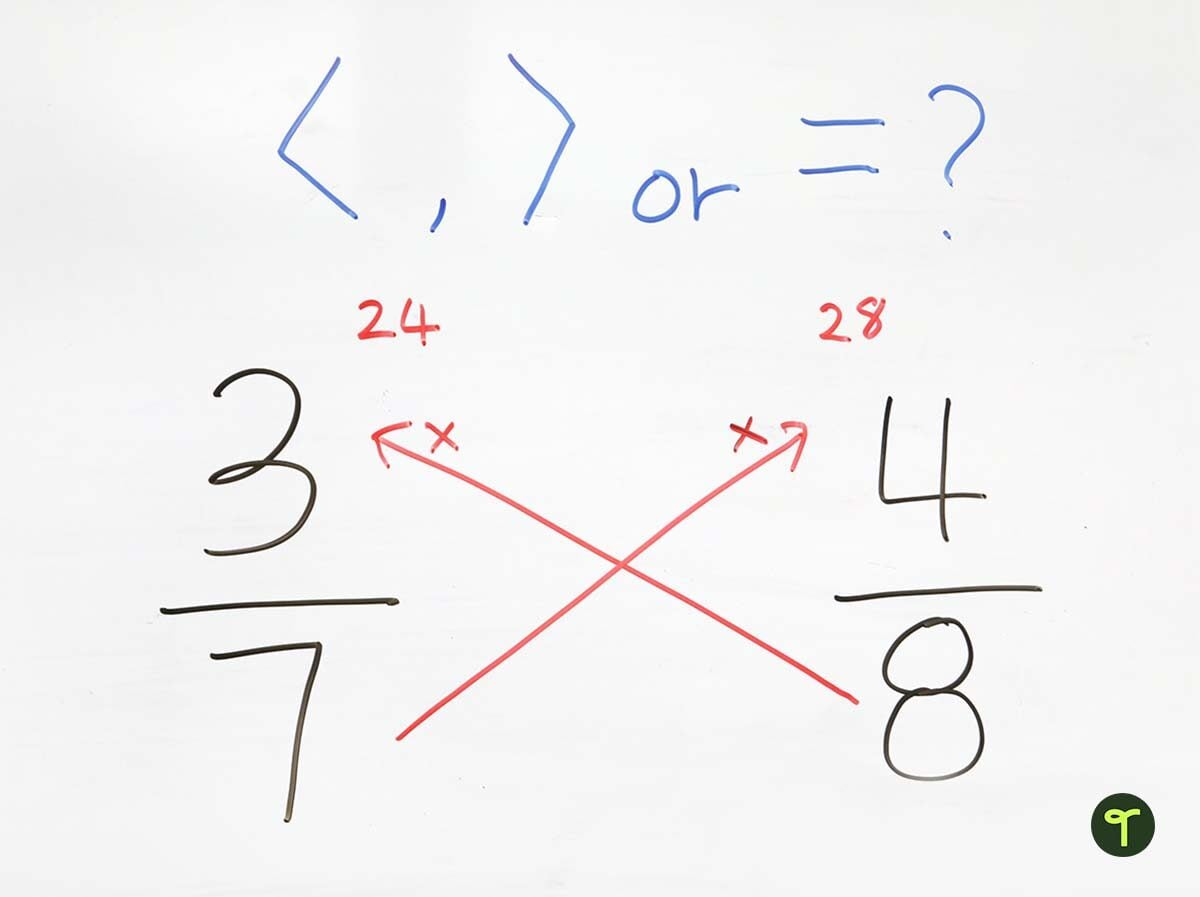The numbers that students get from cross multiplying fractions become the two numerators they would get if they’d gone through the process of creating two ‘like’ fractions. All they need to do now is determine the bigger number of the two!

Print an equivalent fraction poster to serve as a reference point in your classroom.

### Multiplying Double-Digit Numbers by 11

To multiply any two-digit numbers by 11, simply add the digits of the number together. Put the result of your addition between the two digits of your original number.

For example, to quickly find the answer to 26 x 11, start by adding the two digits of the number 26 together to get 2+6=8.

Next, put this new number between the original two digits to get 286. That’s the answer!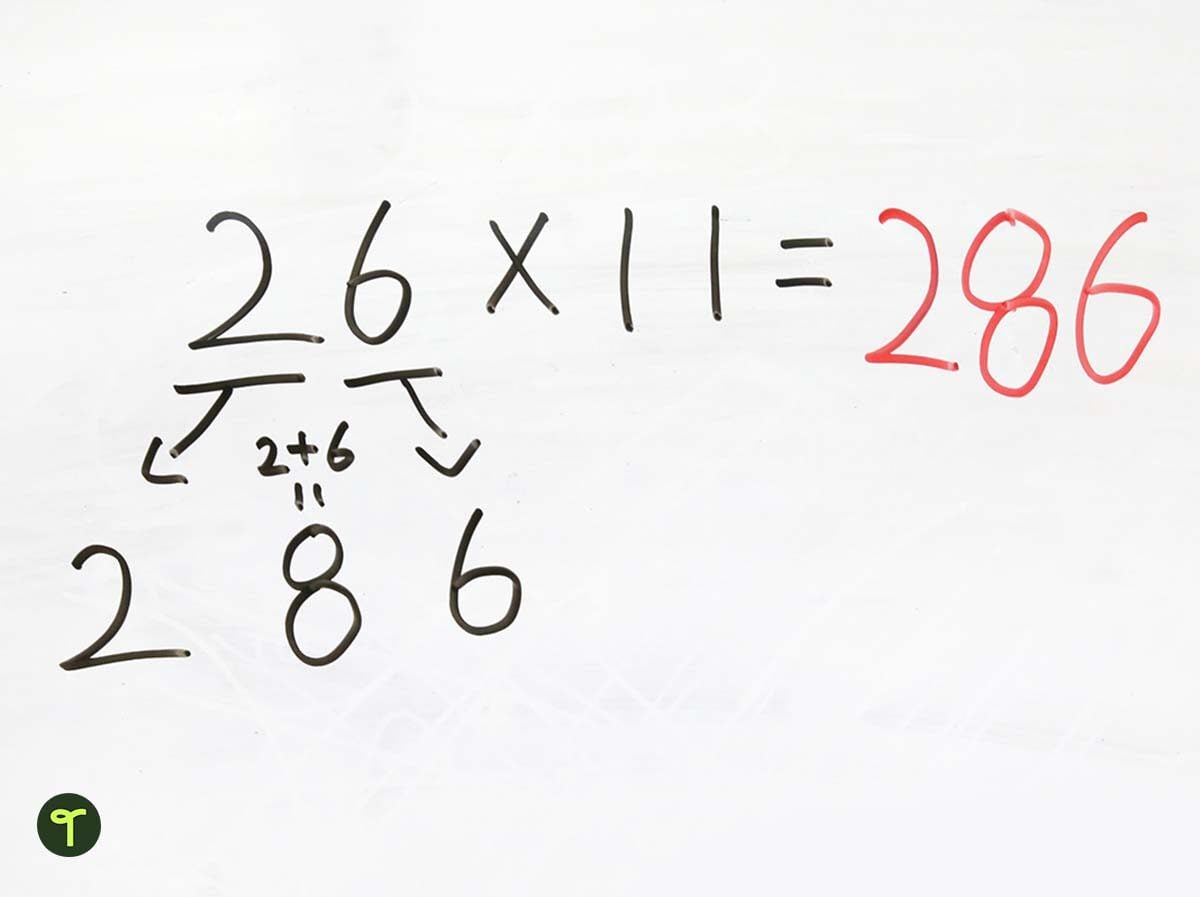But wait a second. What if the sum of the two digits is greater than 9? In that case, carry the 1 over to the tens digit and add the two numbers. For example: 47 x 11 = 517. When you break it down: 4+7 = 11.

### Japanese Multiplication

Have you seen this multiplication trick? It can get tricky with middle numbers, so we’re going to mark this down as something fun to teach at the end of the year!

This trick takes a multiplication problem and turns it into a simple counting problem that can be solved visually. To show this math trick in action, let’s start with a simple multiplication problem. Basically, you are looking at 2 x 3, or 2 groups of 3.

By drawing 2 lines one way and 3 lines the other, you create an intersection of 2 groups of 3. By counting all of the times the lines intersect, you get your answer: 6.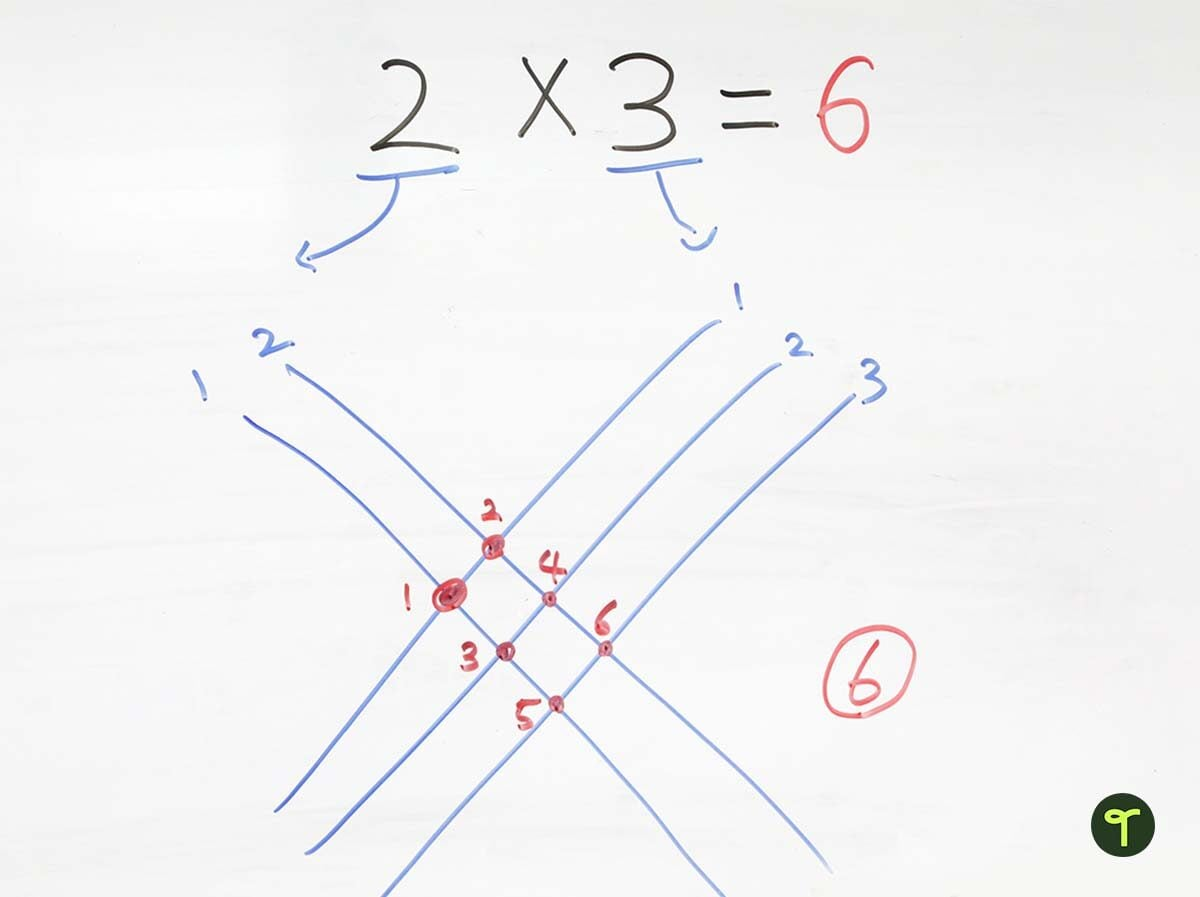Now, let’s try a trickier multiplication problem.

• Each place value number in both numbers requires a set of lines.
• The 1 line for the number 1 in 12 is representing (1) ten.
• The 2 lines (going the same way) represent (2) ones.
• With the number 23, the 2 lines (going the other way) represent the (2) tens and the 3 lines represent the (3) ones.
• You need to make sure that both numbers you are multiplying crossover like the image below.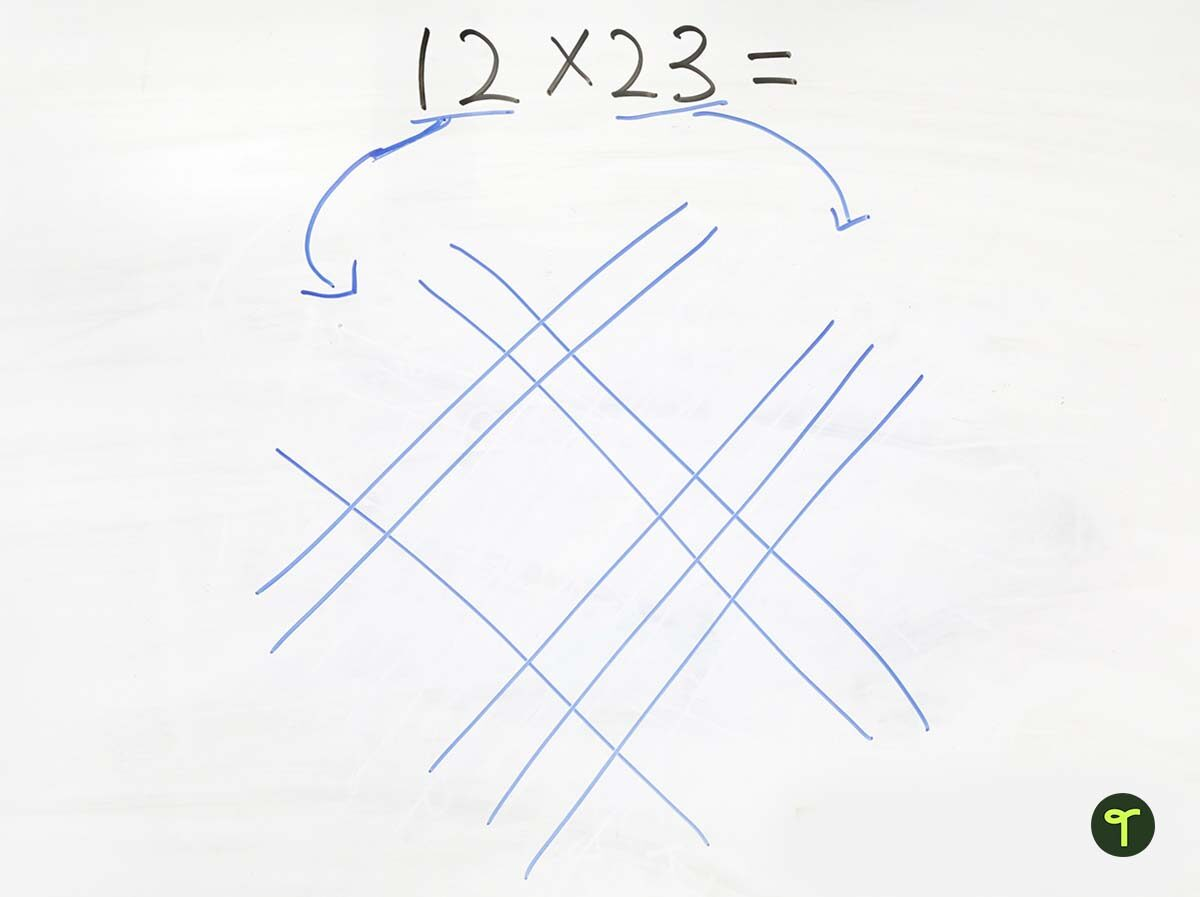On the left corner, put a curved line through the wide spot with no points, and do the same on the right, separating the intersections into three groups.

Finally, count the three groups of intersections, giving you the answer of 276.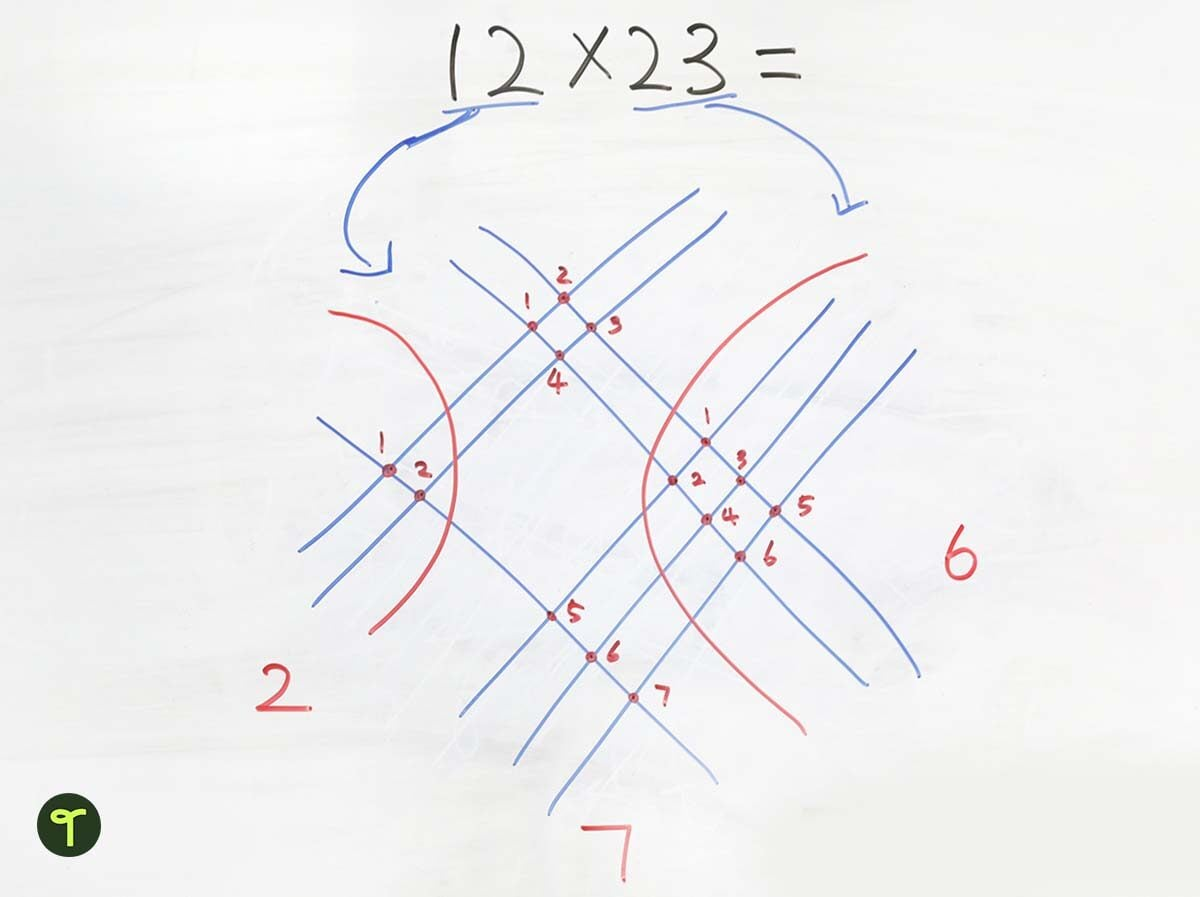### How does Japanese Multiplication work?

Remember, we are looking at the problem 12 x 23.

• Let’s start with the intersections on the far right. There are 6 intersections, which represent 2 ones x 3 ones. So 6 will be in our ones place for the answer.
• On the far left, there are 2 intersections. This set of lines represents the tens in the original numbers used in the problem. So 2 is 20, and 1 is 10, meaning 20 x 10 equals 200. So, our answer will begin with the number 2 in the hundreds place.
• In the top middle section, we have 2 tens x 2 ones which give us 40. Plus, in the bottom middle section, we have 1 ten x 3 ones which give us 30. We have a total of 70 for the tens place.
• All of this gives us a grand total of 276!

#### Check out our full collection of math games, activities, worksheets and more teacher resources ready-made for the classroom!

Banner image via shutterstock/Lorena Fernandez